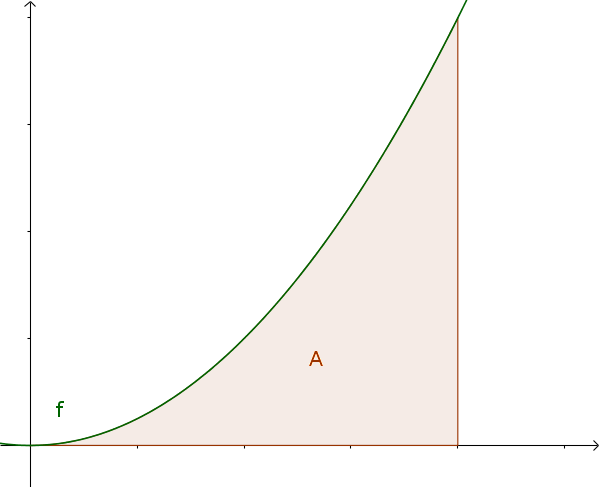Math Definite integral Upper and lower sum

# Upper and lower sum

Integral calculus is used to calculate the area in an interval between the graph of a function and the x-axis.i

### Info

As early as 260 BC, Archimedes developed the partition of an interval method, which forms the origin of integral calculus.

If you want to determine the area now, one partitions the area into vertical rectangles. There are two possibilities:The first partition of the surface is called lower sum and is smaller than the area.This is the upper sum which is larger than the actual area.

$\text{lower sum} \le A \le \text{upper sum}$

!

### Remember

The smaller one sets the distances between the partitions (i.e. the more partitions), the more accurate the result becomes.

### Example

$f(x)=x^2$ in the interval $[0; 1]$

You can now calculate the areas of the rectangles (width is $0.25$ and height is $x^2$) and get the following:

#### Lower sum

$s=0.25\cdot (0^2+0.25^2+0.5^2+0.75^2)$ $=\frac{7}{32}$

#### Upper sum

$S=0.25\cdot (0.25^2+0.5^2+0.75^2+1^2)$ $=\frac{15}{32}$

#### Result

$\frac{7}{32} \le A \le \frac{15}{32}$

With a higher number of partitions, the result becomes more and more accurate. So at a number of 256 partitions:

$0.331\le A\le 0.335$